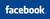FD - Fixed Deposit Compound Interest CalculatorCompound interest Calculator   AllBankingSolutions.com ......our answer to all your banking needsCompound Interest Rate Calculator

Compound Interest Rate Calculator 'gives the Maturity Value' of  the Principal by compounding the interest at the intervals chosen.   In this calculator, you have the option of choosing the compounding frequency as  either as  Monthly or Quarterly or  Half-yearly or Yearly or None.   In India most of the banks have been compounding the interest on quarterly basis, but recently RBI has allowed the same to be compounded even at earlier intervals also.  (In case you wish to calculate the interest for fraction of years at quarterly compounding, please see the link at the end of this article, and go to FD calculator)

The calculator also gives the "Effective Yield", which actually is the return on the deposit in simple interest format.   It depends on the rate of interest and the frequency of compounding.

 Principal Amount (in Rupees) Period of  Deposit  (in years) (fractions not allowed) Rate of Interest (in %) Frequency of Compounding Select Monthly Quarterly HalfYearly Annual None Effective yield % Maturity Amount of the Deposit (Rs.)

The above calculator can also be used for calculation of Simple Interest Rate calculator by chosing the Frequency of Compounding as "None".   This means the calculator will not do compounding of interest and the results will be based on simple interest method.

Click Here For Fixed Deposit Interest Calculator (based on quarterly compounding for any number of months) : FD Calculator (Fixed Deposit Calculator)